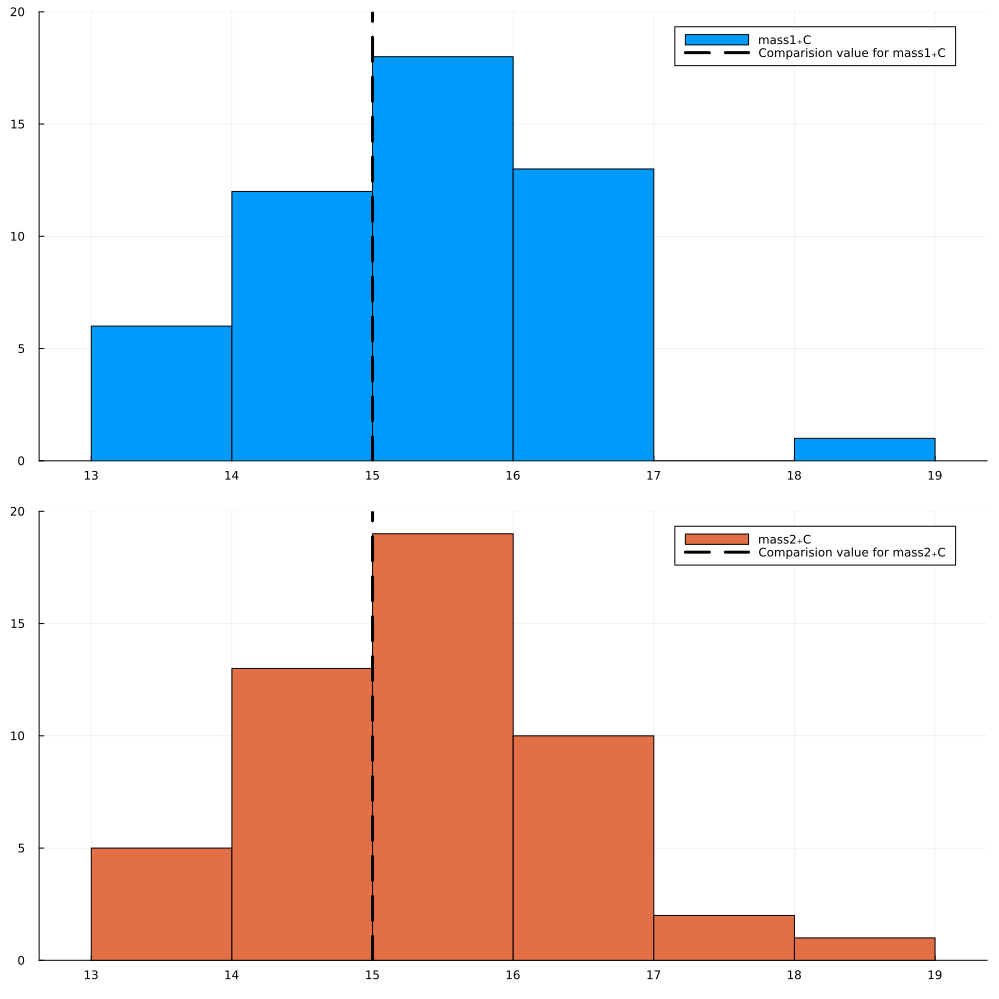# Global Model Calibration of Thermal Conduction

Julia ModelSim Optimizer can be seamlessly integrated with custom components generated through the Modeling Toolkit Standard Library. The thermal conduction model is the first example we are exploring in this integration.

## Thermal conduction model

Here we present an example of integrating the MTK Standard Library with JuliaModelSimOptimizer by using the thermal conduction example. This example demonstrates the thermal response of two masses connected by a conducting element. The two masses have the same heat capacity but different initial temperatures (T1=100 [°C], T2=0 [°C]). The mass with the higher temperature will cool off while the mass with the lower temperature heats up. They will each asymptotically approach the calculated temperature TfinalK that results from dividing the total initial energy in the system by the sum of the heat capacities of each element.

## Julia environment

# Packages used for this example
using JuliaSimModelOptimizer
using OrdinaryDiffEq
using ModelingToolkit
using DataFrames
using ModelingToolkitStandardLibrary.Thermal
using Statistics
using StatsPlots
Plots.GRBackend()

## Model setup

@parameters t

C1 = 15
C2 = 15
@named mass1 = HeatCapacitor(C=C1, T=373.15)
@named mass2 = HeatCapacitor(C=C2, T=273.15)
@named conduction = ThermalConductor(G=10)
@named Tsensor1 = TemperatureSensor()
@named Tsensor2 = TemperatureSensor()

connections = [
connect(mass1.port, conduction.port_a),
connect(conduction.port_b, mass2.port),
connect(mass1.port, Tsensor1.port),
connect(mass2.port, Tsensor2.port),
]

@named model = ODESystem(connections, t, systems=[mass1, mass2, conduction, Tsensor1, Tsensor2])
sys = structural_simplify(model)
prob = ODEProblem(sys, Pair[], (0, 5.0))
sol = solve(prob, Tsit5());
retcode: Success
Interpolation: specialized 4th order "free" interpolation
t: 10-element Vector{Float64}:
0.0
0.12852257621377688
0.4038807431204407
0.7558959430293956
1.2017065981077266
1.7472128926528618
2.411755380896035
3.2154720536986767
4.1946269576983495
5.0
u: 10-element Vector{Vector{Float64}}:
[373.15, 273.15]
[365.27576601244925, 281.0242339875507]
[352.33093980132446, 293.9690601986755]
[341.3999874691451, 304.90001253085484]
[333.2220314449849, 313.07796855501505]
[328.0169841215768, 318.28301587842316]
[325.1570263575137, 321.14297364248625]
[323.8380119351041, 322.4619880648959]
[323.33737373761187, 322.9626262623881]
[323.21409138064587, 323.0859086193541]

## Creating the inverse problem

After generating the solution above, we can convert it into a data frame and generate a Experiment object. This Experiment object can then be fed into the InverseProblem interface. An inverse problem is a collection of trials which define a multi-simulation optimization problem. The solution to an inverse problem are the "good parameters" which make the simulations simultaniously fit their respective data sufficiently well. Next to the model and the trials, the user needs to specify a search space. This is to constrain the optimiser in which values to consider as potential values for the parameter combinations.

data = DataFrame(sol)

experiment = Experiment(data, sys, tspan=(0.0, 5.0))

invprob = InverseProblem(experiment, sys, [mass1.C => (10, 20), mass2.C => (10, 20)]);
InverseProblem for model with one experiment optimizing 2 parameters.


## Generating and visualizing the optimized parameters

Once the inverse problem is defined, we can generate a virtual population of all the parameters and visualize the results! In this example, we are optimizing for 2 parameters simultaneously: the heat capacitances C1, C2.

ps = parametric_uq(invprob, StochGlobalOpt(maxiters=10), sample_size = 50);

plot(ps, layout = (2,1), bins = 8, show_comparision_value = true, max_freq = [20, 20], legend = :best, size = (1000,1000))As we see above, the parameters recovered match the true parameters very well!